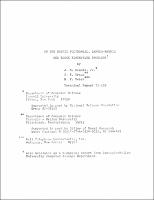## On the Matrix Polynomial, Lamda-Matrix and Block Eigenvalue Problems##### Abstract
A matrix $S$ is a solvent of the matrix polynomial $M(X) \equiv X^{m} + A_{1}X^{m-1} + \cdots + A_{m}$, if $M(S) = \stackrel{0}{=}$, where $A_{1}, X$ and $S$ are square matrices. We present some new mathematical results for matrix polynomials, as well as a globally convergent algorithm for calculating such solvents. In the theoretical part of this paper, existence theorems for solvents, a generalized division, interpolation, a block Vandermonde, and a generalized Lagrangian basis are studied. Algorithms are presented which generalize Traub's scalar polynomial methods, Bernoulli's method, and eigenvector powering. The related lambda-matrix problem, that of finding a scalar $\lambda$ such that $I \lambda^{m} + A_{1} \lambda^{m-1} + \cdots + A_{m}$ is singular, is examined along with the matrix polynomial problem. The matrix polynomial problem can be cast into a block eigenvalue formulation as follows. Given a matrix $A$ of order mn, find a matrix $X$ of order n, such that $AV=VX$, where $V$ is a matrix of full rank. Some of the implications of this new block eigenvalue formulation are considered.
1971-09
##### Publisher
Cornell University
##### Keywords
computer science; technical report
##### Previously Published As
http://techreports.library.cornell.edu:8081/Dienst/UI/1.0/Display/cul.cs/TR71-109
technical report